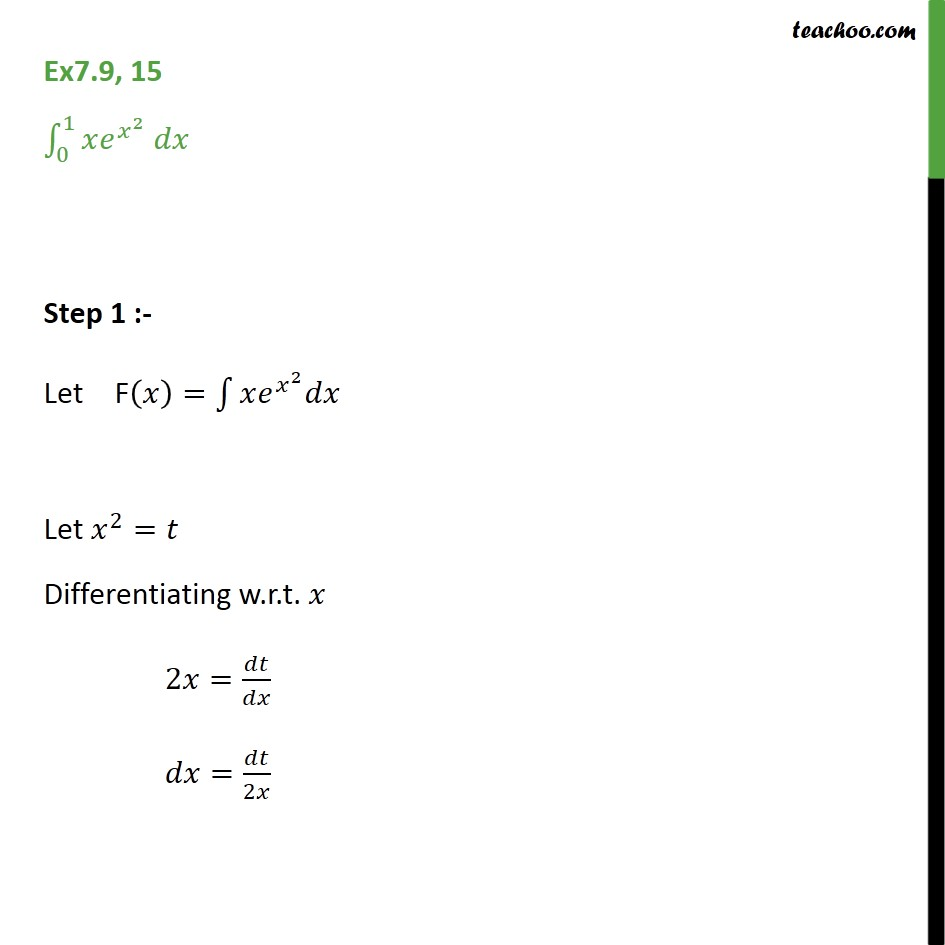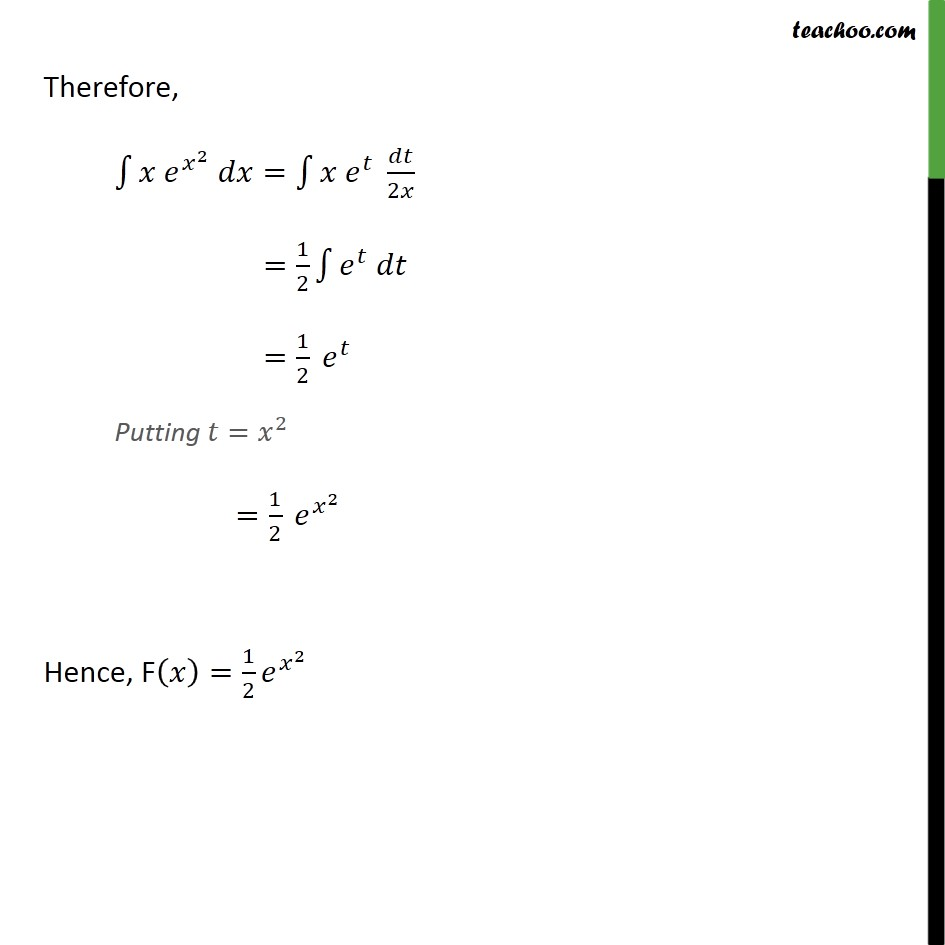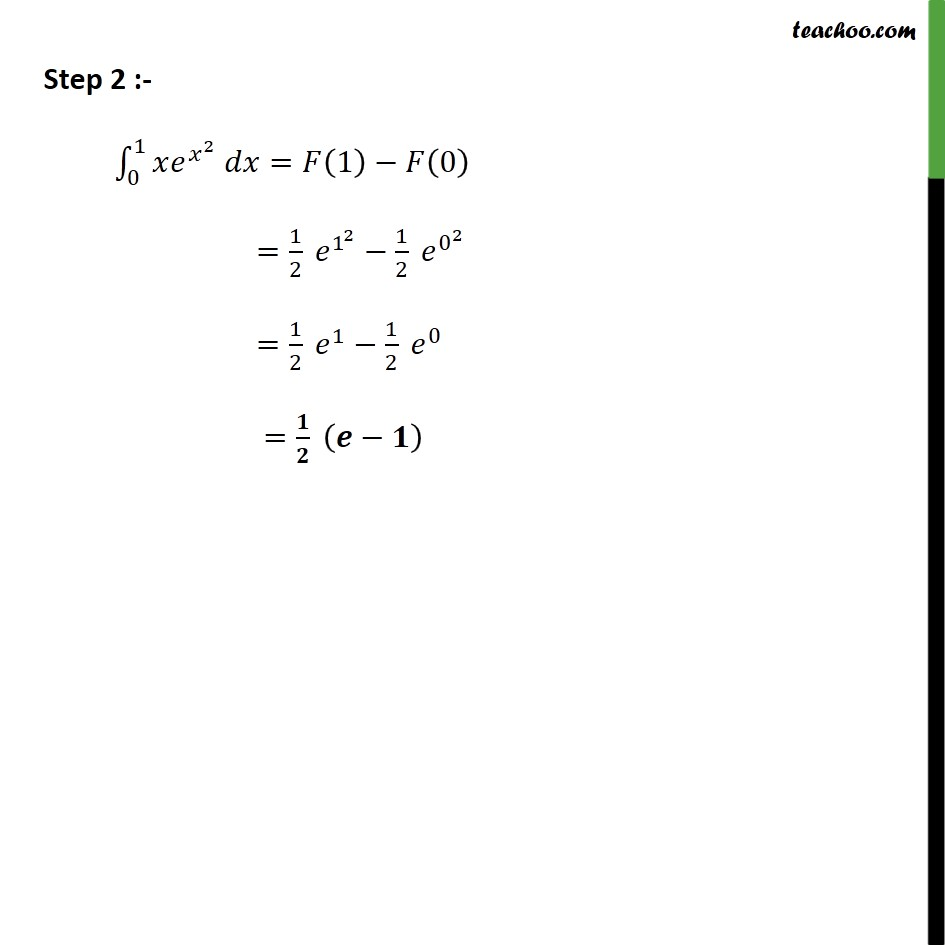Definite Integration - By Substitution

Chapter 7 Class 12 Integrals
Concept wiseIntroducing your new favourite teacher - Teachoo Black, at only ₹83 per month

### Transcript

Ex7.9, 15 _0^1 ^ ^2 Step 1 :- Let F( )= 1 ^( ^2 ) Let ^2= Differentiating w.r.t. / ( ^2 )= / 2 = / = /2 Therefore, 1 ^( ^2 ) = 1 ^ /2 =1/2 1 ^ =1/2 ^ Putting = ^2 =1/2 ^( ^2 ) Hence F( )=1/2 ^( ^2 ) Step 2 :- _0^1 ^ = (1) (0) =1/2 ^(1^2 ) 1/2 ^(0^2 ) =1/2 ^1 1/2 ^0 =1/2 ( 1)